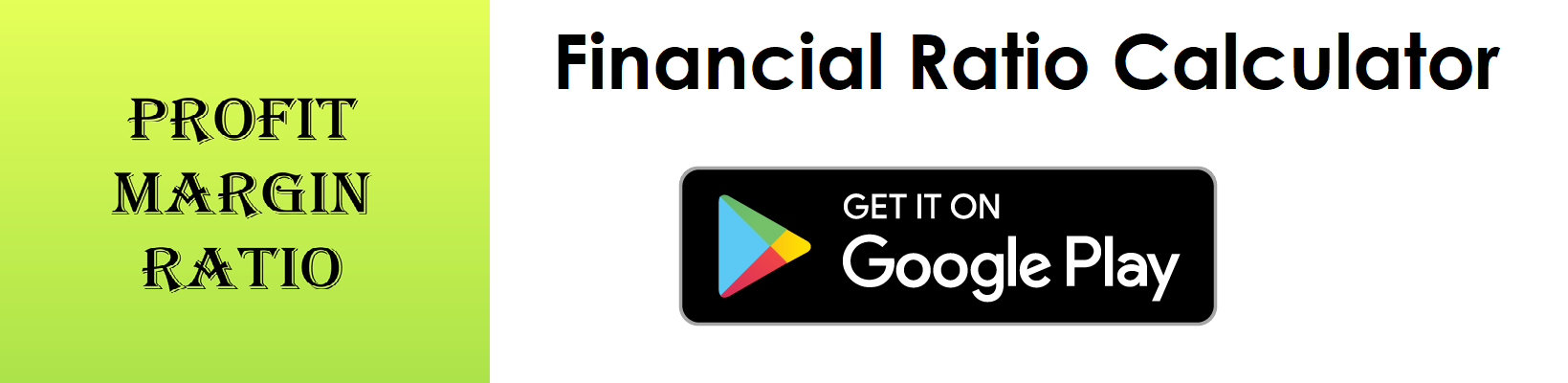# Current Ratio Calculator

Key in the Current Assets and Current Liabilities Values from your Balance Sheet to the respective fields given below and then click Calculate to get the desired result.

##### Current Ratio Calculator - Glossary:
Using our current ratio calculator, helps to compare, measure, understand the overall health of the business.

##### Current Ratio:
Shows the proportion of current assets to current liabilities. In simple words, it measures a company's ability to pay the short-term liabilities with its current assets.

##### How to calculate?
Formula: Current Ratio = Current Assets/Current Liabilities.
This is a balance sheet component; the values are commonly stated against current assets and current liabilities.

##### Current Assets:
This is a balance sheet component - cash, cash equivalents, account receivables, merchandise inventory and marketable securities can easily be converted into cash in the short-term.

##### Current Liabilities:
This is a balance sheet component - accounts payable, tax payable (sales, payroll and other taxes) that are due in short-term.

##### Example:
Current ratio for a company with a total current assets of \$200,000 and current liabilities of \$155,000 is 1.29 : 1. It means \$1.29 of current assets are available to cover each \$1 of its current liabilities.

# Download our Mobile Apps - today.